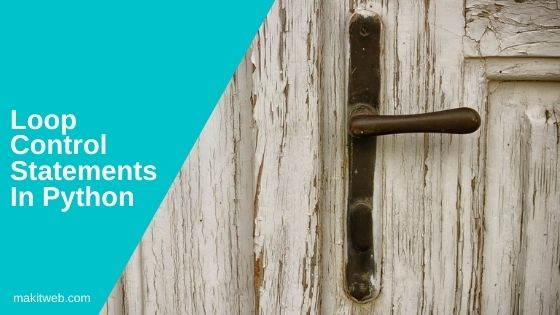# Loop control statements in Python

Loop control statements are used to handle the flow of the loop e.g. terminate the loop or skip some block when the particular condition occurs.

There are the following types of loop control statements in Python –

1. break
2. continue
3. pass## 1. break

`break` statement is used to terminate the loop when the specified condition arises. This statement is also useful to terminate the infinite loop and resume execution to the next statement.

Syntax –

```break
```

Example –

break statement with while loop

```num = 1

while num<=5 :
print("num : ",num)
if num == 3
break
num+=1

print("Bye")
```

Output

```num : 1
num : 2
num : 3
Bye
```

break statement with for loop

```languages = ["C","Python","PHP","JAVA","Javascript","jQuery"]

for lang in languages:
if lang == "JAVA":
break
print(lang)

print("Bye")
```

Output

```C
Python
PHP
Bye
```

Terminating the infinite loop

```num = 1

while 1:
if num == 5:
break
print("num : ",num)
num += 1

print("Bye")
```

Output

```num : 1
num : 2
num : 3
num : 4
Bye
```

## 2. continue

This statement skips the block of code that executes after it and returns the control to the beginning of the loop. You can use it in both `while` and `for` loop.

Syntax –

```continue
```

Example –

continue statement with while loop

```num = 1

while num<=10 :
num += 1
if num == 3 or num == 5
continue

print("num : ",num)

print("Bye")
```

Output

```num : 2
num : 4
num : 6
num : 7
num : 8
num : 9
num : 10
num : 11
Bye
```

continue statement with for loop

```languages = ["C","Python","PHP","JAVA","Javascript","jQuery"]

for lang in languages:
if lang == "JAVA":
continue
print(lang)

print("Bye")
```

Output

```C
Python
PHP
Javascript
jQUery
Bye
```

## 3. pass

`pass` statement is a little different from previous statements when it executes nothing happens.

It is useful when you are implementing a conditional statement or defining method in a program but at the current time, you don’t decide what it will do or a block of code is no longer required in the program.

Syntax –

```pass
```

Example –

```num = 1

while num<=5 :
print("num : ",num)
if num == 3
print("pass statement block")
pass
num+=1

print("Bye")
```

Output

```num : 1
num : 2
num : 3
pass statement block
num : 4
num : 5
Bye
```